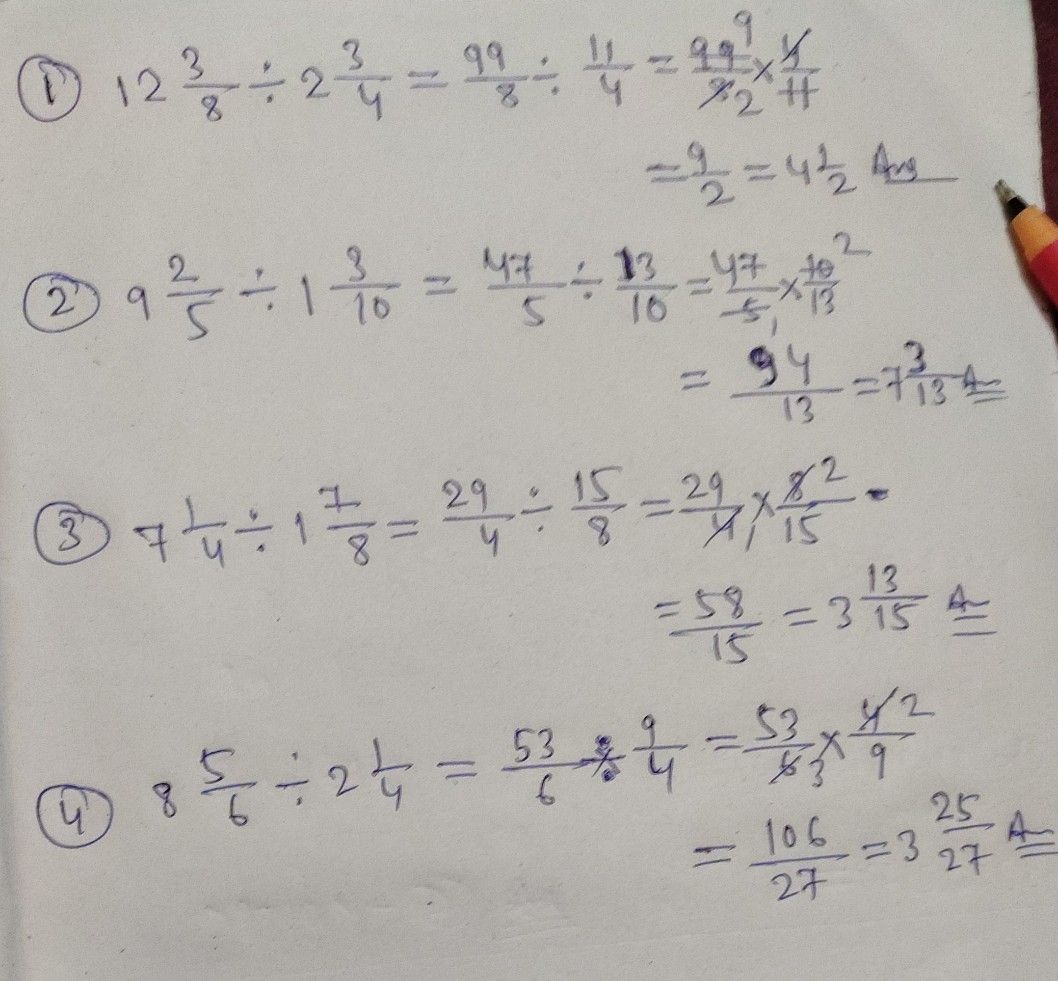Symbol
ProblemLearning Task $2$ Find the quotient of the given fractions below. Write your solution in your notebook. $+2\dfrac {2} {4}$ $21\dfrac {1} {4}$ $41\dfrac {7} {2}$ $+$ $2\dfrac {10} {21}$ $7$ $3$ $+3\dfrac {1} {2}$ $2$ $-$ $7$ $2\dfrac {1} {4}$ $12\dfrac {2} {2}+3\dfrac {13} {24}$ 8. $14\dfrac {2} {3}+2\dfrac {2} {5}$
1st-6th grade
Calculus
Search count: 106
Question content
help me again to my work:(
SolutionQanda teacher - Ranjeet